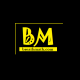# What is the practical application of congruence nowadays?

Answer to the comment in Congruences, by dwightisebia

First of all I liked your question. Actually it gave me an idea of adding real life applications of mathematical concepts to my site! Thank you!

Q: What is the practical application of congruence nowadays?
Ans:

Two objects are congruent if they have the same dimensions and shape. Clearly, you can think of it as meaning ‘equal’.

When any object is kept in front of the mirror, that mirror image is congruent to the real image.

When two objects or shapes are said to congruent then all corresponding angles and sides also congruent.
For example:Real life examples are,

• cigarettes in a packet are congruent to one another.
• Giant wheels or ferris wheels.
• Pages of one books are congruent to one another and etc

## 6 thoughts on “What is the practical application of congruence nowadays?”

1.dwightisebia says:

But how to relate the modulo to congruence in real life. In other words, how to perceive the “equality” of 27 and 47 in relation to to the modulo 10?

Liked by 1 person

1.breathmath says:

Hahaha! 27 and 47! Haha!

The modulo operation is taking remainder when dividing. For example 5 mod 3 = 2, means 2 is the remainder when divided 5 by 3. This can be used in everyday math calculations 😀

Let x and y be two kids, x had 27 rs with him & y had 47 rs with him. While teaching them money calculations their mamma told them to make groups of 10rs from the money they have!
Accordingly, x makes group of 2 and he finds 7rs extra with him that he cannot group-up!
Mathematical expression of this is, 27 ≡ 7 (mod 10) ——–(1)
Similarly, y makes group of 4 and he also finds 7rs extra with him. Mathematical expression of this is, 47 ≡ 7 (mod 10)———(2)
From (1) and (2)
27 ≡ 47(mod 10) as they get same remainder i.e., 7 when divided by 10.
Hope you got! 27 and 47! 😀

If not, I can explain Modulo operation by taking example as CLOCKS…

Mathematics is abstract! You cannot find numbers, mathematical symbols in nature. Mathematics is used in predicting and controlling real objects and events, from calculating a shopping bill to sending rockets to other planets!

Liked by 1 person

1.dwightisebia says:

Clear!

Liked by 1 person

2.breathmath says:

Thank you!

Like

3.dwightisebia says:

I sometimes use math principles in poetry

Liked by 1 person

4.breathmath says:

That’s great .. when your posts are related to math , tag me up!

Like